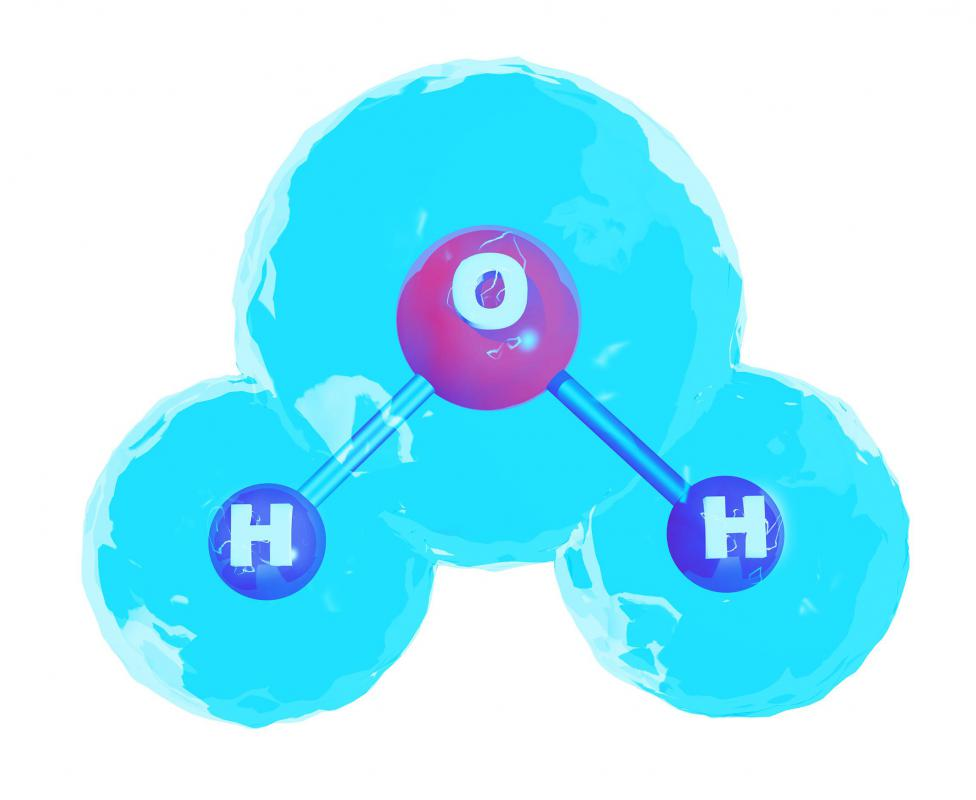# What Is Solute Concentration?

Paul Reed

Solute concentration is a term used to describe mixtures, and defines how much of one substance, called the solute, is dissolved in another, referred to as the solvent. There are a number of ways to describe concentration, depending on need, and can involve weight, volume or molecular mass. The concentration of mixtures is important because many chemical reactions are dependent on having the correct amount of reactants to complete the reaction.

Perhaps the easiest way to describe solute concentration is by weight. A person wishing to make a consistent mixture of salt in water might weigh both materials and record the results. Weighing the same amounts repeatedly can then reproduce the salt-water concentration, which will provide equivalent mixtures. A mixture made using this method is usually called a weight percent mixture, which defines that weights were used rather than volume.A molecule of water contains two atoms of hydrogen and one oxygen.

Weight per volume is also a common measurement of solute concentration. An amount of solute is first weighed using a measuring scale, then added to a container. The solvent is then added to a volume mark on the container, resulting in a known volume of mixture. The resulting mixture is defined as weight per volume, such as pounds per gallon or grams per liter.

Volume solute concentration is possible if both materials are liquids, but is not used for solids dissolved in liquids. A marked container is used to measure the volume of the solute and solvent, then they are mixed. The concentration is referred to as a volume percent to identify that both materials are present in volume, rather than weight.

For laboratory use, molar or molal concentrations are often used to accurately determine the amount of material available for chemical reactions. The moles of a solute equal the weight of the material divided by the molar mass, which is determined mathematically from the periodic table of elements. For example, a molecule of water contains two atoms of hydrogen and one oxygen. The molar mass of hydrogen and oxygen can be found on any published periodic table.

Once found, the molar mass of the molecule is determined by adding the molar mass of oxygen to two masses of hydrogen, which equals the atoms present in a water molecule. Molar mass has no units, but is often published as grams per mol; this can be pounds per mol if the molar masses are pounds. The number of moles is often determined by the chemical reaction itself, since products react according to their molar masses when the molecules combine.

To determine how much solute to add in a molar mixture, the number of moles needed is multiplied by the molar mass to obtain a weight. This weight is added to a container, and a known amount of solvent volume is added, typically a liter or gallon to obtain a consistent concentration. The result is a moles per volume ratio, which is called molar solute concentration. Adding the same weight to a known weight of solvent gives the molal concentration.

## You might also Like